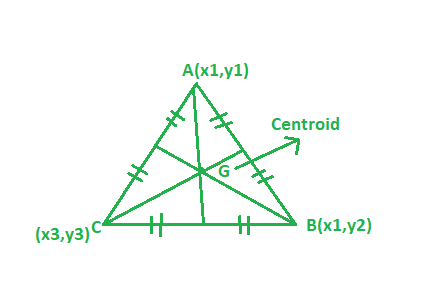# Program to find the Centroid of the triangle

• Last Updated : 22 Mar, 2021

Given the vertices of the triangle. The task is to find the centroid of the triangle:
Examples:

Input: A(1, 2), B(3, -4), C(6, -7)
Output: (3.33, -3)

Input: A(6, 2), B(5, -9), C(2, -7)
Output: (6.5, -9)Approach:Suppose if the vertices of a triangle are (x1, y1) (x2, y2) (x3, y3) then centroid of the triangle can be find from the following formula:## C++

 // CPP program to find the centroid of triangle#include using namespace std; // Driver codeint main(){    // coordinate of the vertices    float x1 = 1, x2 = 3, x3 = 6;    float y1 = 2, y2 = -4, y3 = -7;     // Formula to calculate centroid    float x = (x1 + x2 + x3) / 3;    float y = (y1 + y2 + y3) / 3;     cout << setprecision(3);    cout << "Centroid = "         << "(" << x << ", " << y << ")";     return 0;}

## Java

 // Java program to find the centroid of triangleimport java.util.*;import java.lang.*; class GFG{     // Driver codepublic static void main(String args[]){    // coordinate of the vertices    float x1 = 1, x2 = 3, x3 = 6;    float y1 = 2, y2 = -4, y3 = -7;     // Formula to calculate centroid    float x = (x1 + x2 + x3) / 3;    float y = (y1 + y2 + y3) / 3;     //System.out.print(setprecision(3));    System.out.println("Centroid = "        + "(" + x + ", " + y + ")");}} // This code is contributed// by Akanksha Rai(Abby_akku)

## Python 3

 # Python3 program to find# the centroid of triangle # Driver code    if __name__ == "__main__" :     # coordinate of the vertices    x1, x2, x3 = 1, 3, 6    y1, y2, y3 = 2, -4, -7         # Formula to calculate centroid    x = round((x1 + x2 + x3) / 3, 2)    y = round((y1 + y2 + y3) / 3, 2)     print("Centroid =","(",x,",",y,")") # This code is contributed by ANKITRAI1

## C#

 // C# program to find the// centroid of triangleusing System; class GFG{     // Driver codestatic public void Main (){     // coordinate of the vertices    float x1 = 1, x2 = 3, x3 = 6;    float y1 = 2, y2 = -4, y3 = -7;         // Formula to calculate centroid    float x = (x1 + x2 + x3) / 3;    float y = (y1 + y2 + y3) / 3;         //System.out.print(setprecision(3));    Console.Write("Centroid = " +                  "(" + x + ", " + y + ")");}} // This code is contributed// by RaJ

## PHP

 

## Javascript

 
Output:
Centroid = (3.33, -3)

My Personal Notes arrow_drop_up